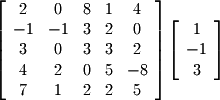Matrix/scalar multiplication is also analogous to vector/scalar multiplication, but matrix/matrix multiplication is somewhat more complicated. The greatest difficulty students have with matrix multiplication is that it can be a long process and therefore tedious. Since lots of arithmetic has to be done, there are ample opportunities to make simple mistakes. The process itself is straightforward. Take an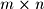matrix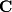which represents the product of an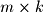matrix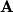with a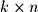matrix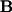. An entry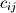is the dot product of theth row of(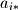) with the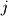th column of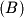(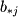). Using this definition iteratively for all the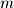rows ofand all the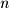columns ofwill generate. This definition constrains the resulting dimensions of. Since the dot product is used, the number of columns inmust equal the number of rows inso that the dotted vectors will have equal dimensions (in this case, both are equal to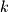). Also,will have the same number of rows as() and the same number of columns as(). This is easy to remember: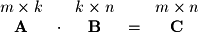Additionally, matrix multiplication is not commutative: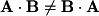The reason is obvious when one looks back at the original definition. It is, however, both associative and distributive: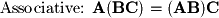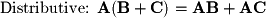Excercise 3-2.
For each of the following matrix pairs, state whether or not the multiplication is possible (do not perform the multiplication). If the multiplication is valid, state the resulting dimensions:
1.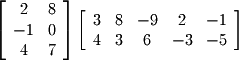2.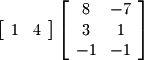3.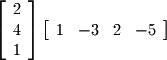4.